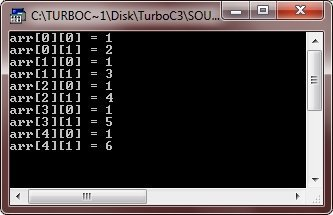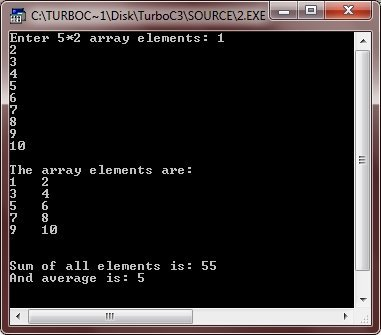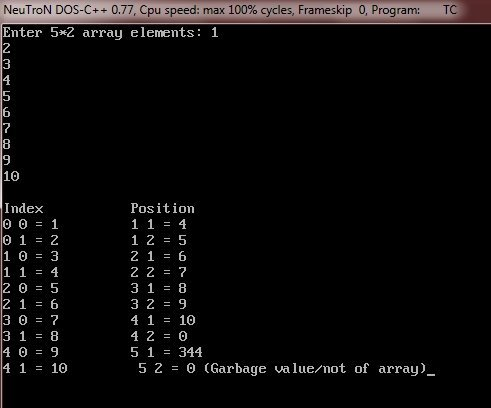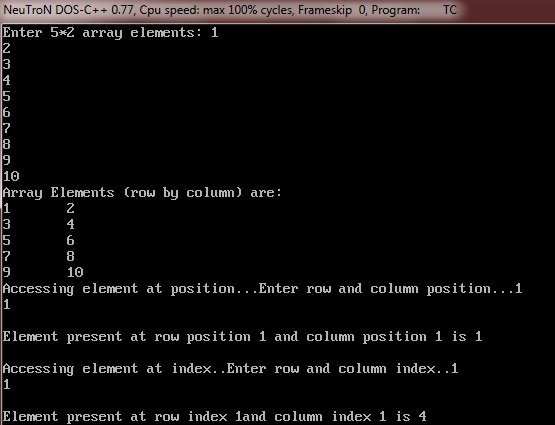# C++ Two Dimensional Array

A two-dimensional array is an array in which each element is itself a 1-D array.

## Declare Two Dimensional Array in C++

Here is the general form to declare two dimensional array in C++

`data_type array_name[row_size][column_size];`

Here data_type is any valid C++ data type, array_name is the name of the array, row_size and column_size is the dimension of the two dimensional array. If row size is 5 and columns size is 2, then the dimension of the two dimensional array will be 5*2, total size becomes 10. Therefore, 10 element holds this two-dimensional array. Here is an example declaring two dimensional array named arr with dimension 5*2

`int arr;`

## Initialize Two Dimensional Array in C++

Here is the general form to initialize values to two dimensional array in C++

`data_type array_name[row_size][column_size] = { {comma_separated_value_list} };`

Here is an example, declaring and initializing values to two dimensional array named arr of type int, containing 5 row and 2 column i.e., dimension of this array is 5*2

`int arr = { {1, 2}, {3, 4}, {5, 6}, {7, 8}, {9, 10} };`

## C++ Two Dimensional Array Example

Here are some C++ programs, demonstrating two dimensional array in C++

```/* C++ Two Dimensional Array */

#include<iostream.h>
#include<conio.h>
void main()
{
clrscr();
int arr = { {1, 2}, {1, 3}, {1, 4}, {1, 5}, {1, 6} };
int i, j;
for(i=0; i<5; i++)
{
for(j=0; j<2; j++)
{
cout<<"arr["<<i<<"]["<<j<<"] = "<<arr[i][j]<<"\n";
}
}
getch();
}```

Here is the sample output of the above C++ program:Here is another C++ program, also demonstrating two dimensional array in C++

```/* C++ Two Dimensional Array */

#include<iostream.h>
#include<conio.h>
void main()
{
clrscr();

int arr;
int i, j;
int sum=0, avg=0;

cout<<"Enter 5*2 array elements: ";
for(i=0; i<5; i++)
{
for(j=0; j<2; j++)
{
cin>>arr[i][j];
sum = sum + arr[i][j];
}
}
cout<<"\nThe array elements are: \n";
for(i=0; i<5; i++)
{
for(j=0; j<2; j++)
{
cout<<arr[i][j]<<"   ";
}
cout<<"\n";
}
cout<<"\n\nSum of all elements is: "<<sum;
avg = sum/10;
cout<<"\nAnd average is: "<<avg;

getch();
}```

Below is the sample run of the above C++ program:Below is one more C++ program on two dimensional array:

```/* C++ Two Dimensional Array */

#include<iostream.h>
#include<conio.h>
void main()
{
clrscr();

int arr;
int i, j, position, index;
cout<<"Enter 5*2 array elements: ";
for(i=0; i<5; i++)
{
for(j=0; j<2; j++)
{
cin>>arr[i][j];
}
}
cout<<"\nIndex\t\tPosition";
for(i=0; i<5; i++)
{
for(j=0; j<2; j++)
{
cout<<"\n";
cout<<i<<" "<<j<<" = "<<arr[i][j]<<"         ";
cout<<i+1<<" "<<j+1<<" = "<<arr[i+1][j+1];
}
}
cout<<" (Garbage value/not of array)";

getch();
}```

Here is the sample run of this C++ program:Let's take one more C++ program, for complete understanding on two dimensional array in C++

```/* C++ Two Dimensional Array */

#include<iostream.h>
#include<conio.h>
void main()
{
clrscr();

int arr;
int i, j;
int row_position, col_position;
int row_index, col_index;

cout<<"Enter 5*2 array elements: ";
for(i=0; i<5; i++)
{
for(j=0; j<2; j++)
{
cin>>arr[i][j];
}
}
cout<<"Array Elements (row by column) are:\n";
for(i=0; i<5; i++)
{
for(j=0; j<2; j++)
{
cout<<arr[i][j]<<"\t";
}
cout<<"\n";
}

cout<<"Accessing element at position...Enter row and column position...";
cin>>row_position;
cin>>col_position;
cout<<"\nElement present at row position "<<row_position;
cout<<" and column position "<<col_position<<" is "<<arr[row_position-1][col_position-1];
cout<<"\n\nAccessing element at index..Enter row and column index..";
cin>>row_index;
cin>>col_index;
cout<<"\nElement present at row index "<<row_index;
cout<<"and column index "<<col_index<<" is "<<arr[row_index][col_index];

getch();
}```

Here is the sample run of the above C++ program:### More Examples

Here are some more C++ programs listed on two and three dimensional array, that you may like:

Tools
Calculator

Quick Links
Signup - Login - Give Online Test